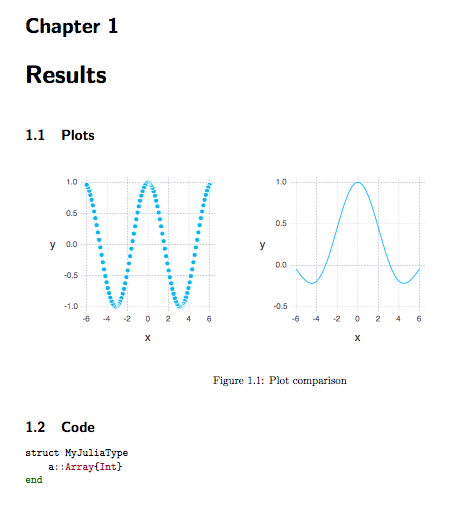36

9

12

3

# LaTeX

This package allows to construct LaTeX documents programmatically.

## Installation

It is assumed that you have `pdflatex` installed. You can then install `LaTeX.jl` like this:

``````]add LaTeX
``````

To be able to use code blocks with syntax highlighting, please install Pygments: `easy_install -U Pygments`.

## Example

``````using LaTeX

x = range(-6,6; length=100)

g = Image(7, 7, plot(x = x, y = sin.(x) ./ x, Geom.line))
w = Image(7, 7, plot(x = x, y = cos.(x)))

# needs pygments to be installed
c = Code("""
struct MyJuliaType
a::Array{Int}
end
""")

openpdf(report(
Section("Results", [
Section("Plots", Figure("Plot comparison",Tabular([w,g]))),
Section("Code", c)
])))
``````## Available functions

`content` can always be either a single item or an array of items.

• `latex = report(content)` assembles the LaTeX file
• `latex = document(content)` gives more control over the look and feel of the document. See here for more.
• `openpdf(latex)` compiles the LaTeX file and tries to open it
• `writepdf(latex, filename)` compiles the LaTeX file and save it to the destination provided
• `Section(title, content)` creates a new section. A section is automatically translated to a Linux chapter, section or subsection according to its nesting
• `Figure(caption, content)`
• `Table(caption content)`
• `Tabular(content)`
• `Code(content)`
• `TOC()` indicates a table of contents
• `Abstract(content)` defines an abstract for the document
• `Image(height, width, Array or Winston.FramePlot or Gadfly.Plot)`, where the array can be either of size `(m,n,1)` or RGB `(m,n,3)`, with the values in the range `0..1`

To define a custom document, use the `document` function, in combination with the following declarations, some of which can be omitted:

• `DocumentClass("article", ["11pt", "letterpaper"])` declares the document class of the file. Settings are passed through a vector as the second parameter.
• `Title("A LaTeX Library for Julia")` declares the title of the file.
• `Date(1999, 12, 31)` declares the date of the file. Note that this is the same `Date` as in standard Julia.
• `Author("John Smith")` declares the author of the file.

For example, the following is a minimal document:

``````document([
DocumentClass("article", ["11pt", "letterpaper"]),
Date(2015, 11, 23),
Title("A LaTeX Library for Julia"),
Author("John Smith"),
Section("Code", [Code("""# minimal example""")])])
``````

## Todos

• make preamble configurable
• adapt `openpdf` to linux

01/04/2015

3 days ago

7 commits